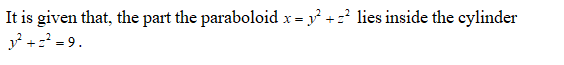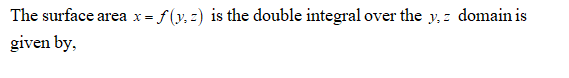# Find the area of the surface.The part of the paraboloid x = y^2 + z^2 that lies inside the cylinder y^2 + z^2 = 9.

Question

Find the area of the surface.

The part of the paraboloid x = y^2 + z^2 that lies inside the cylinder y^2 + z^2 = 9.

check_circleExpert Solution
Step 1Step 2Step 3

Obtain the partial derivatives wi...

### Want to see the full answer?

See Solution

#### Want to see this answer and more?

Solutions are written by subject experts who are available 24/7. Questions are typically answered within 1 hour*

See Solution
*Response times may vary by subject and question
Tagged in

### Calculus